﻿ Mathematical Modeling of Electricity Hybrid Systems Based on Wind, Sun Radiation and Micro Hydro Systems with Efficiency Control Strategy Using Photocell and Motion Sensor or Wifi SwitchApplied Mathematics

p-ISSN: 2163-1409    e-ISSN: 2163-1425

2022;  12(1): 1-7

doi:10.5923/j.am.20221201.01

Received: Feb. 8, 2022; Accepted: Mar. 14, 2022; Published: Mar. 24, 2022### Mathematical Modeling of Electricity Hybrid Systems Based on Wind, Sun Radiation and Micro Hydro Systems with Efficiency Control Strategy Using Photocell and Motion Sensor or Wifi Switch

Tiryono Ruby1, Machudor Yusman2

1Department of Mathematics, Lampung University, Bandar Lampung, Indonesia

2Department of Computer Science, Lampung University, Bandar Lampung, Indonesia

Correspondence to: Tiryono Ruby, Department of Mathematics, Lampung University, Bandar Lampung, Indonesia.
 Email: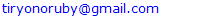Abstract

This paper constructs a mathematical model of a hybrid electric energy system based on renewable energy sources and the reliability of photocell efficiency control strategies, motion sensor and or wi-fi switches in response to fluctuating demand loads. By combining these models and strategies, it is hoped that they can accommodate the phenomenon of the electricity supply system and fluctuations in demand loads that must be met together so that the actual optimal conditions in system operation can be achieved. Thus the system design information obtained from this study can be more accurate and precise so that in making decisions on the procurement of a hybrid system unit, the required power source equipment can be of higher quality and more accurate, especially in this pandemic era.

Keywords: Mathematical model, Hybrid, Renewable sources, Switching

Cite this paper: Tiryono Ruby, Machudor Yusman, Mathematical Modeling of Electricity Hybrid Systems Based on Wind, Sun Radiation and Micro Hydro Systems with Efficiency Control Strategy Using Photocell and Motion Sensor or Wifi Switch, Applied Mathematics, Vol. 12 No. 1, 2022, pp. 1-7. doi: 10.5923/j.am.20221201.01.

### 1. Introduction

Along with population growth and today's developments, the need for electricity today has increased to become a very vital need in the wheel of life, not only in big cities but in remote villages, plantation areas or small islands-even in dire need of electricity . The strategy of the generator unit system (GUS) in procuring electricity sources for electrification has been widely known. However, to maintain a consistent energy supply in this way, it turns out that a lot of the energy produced is wasted when the load that must be met decreases. Unfortunately the generator unit system is not able to store the remaining electrical energy so that a lot of electrical energy is wasted, especially when the output from the generator exceeds the demand load. Operating the genset unit at a low power level for an extended period of time can result in scaling of the engine block cylinder of the generator set. These conditions require special treatment and will cost a higher treatment than regular treatment. The benefits of having electricity facilities include lighting, entertainment, communication, information, industry, irrigation, transportation, security, safety, defense and or research support facilities.
The GUS system is a system for supplying electricity sources that are commonly used in areas that have not been reached by the electricity network of the State Electricity Company . The consideration is that the equipment needed to make the system is easy to obtain, inexpensive, and easy to install. In addition, diesel generators are relied upon to provide a relatively consistent supply of electrical energy. However, to maintain a consistent supply of electrical energy will result in an increase in operational costs, especially for the expenditure of fuel costs that must be provided to keep the generator set in operation. This condition is exacerbated by the high price of fuel in remote locations/plantations or small islands, because the fuel must be imported from outside the area which is quite far away.
Another drawback of the GUS system is its low efficiency. This system is not able to store the remaining electrical energy so that a lot of electrical energy is wasted, especially when the output from the generator exceeds the demand load. If the GUS is operated at an output level 40% lower than maximum capacity, for example during the day, it may reduce the amount of fuel used. However, by operating the GUS lower than the maximum capacity, a lot of electrical energy cannot be produced than it should be. The loss of electrical energy that is not produced as a result of not being utilized optimally from a GUS system is not proportional to the decrease in the amount of fuel used. In fact, the demand load on the system fluctuates so that the GUS rarely operates at maximum power capacity for a sufficiently long period of time.
Another problem in using the GUS system is in terms of maintenance costs. Broadly speaking, maintenance costs depend on how the diesel generator is operated. Generator damage generally occurs in the heating phase, when the generator is started from cold conditions. Frequent switching on and off of the generator should be avoided, and once the generator is turned on it should reach standard operating temperatures and maintain this condition for as long as possible. In fact, this method is often used to adjust the output level or the length of operation time of the GUS according to the demand load. Operating the generator set at a low power level for an extended period of time can result in scaling of the generator engine block cylinder. These conditions require special treatment and will cost a higher treatment than regular treatment .
One alternative to procuring an efficient power source for electrification with relatively low operating costs is the design of a wind, solar and micro hydro-based hybrid system with an operational strategy of controlling efficiency using photocell, motion sensor and or wifi android os. The system is used to procure a power source that has the ability to store the remaining electrical energy and the ability to switch when the load to be fulfilled fluctuates up or down. Therefore, to accommodate the procurement of efficient and renewable or environmentally friendly electricity sources together, from the applied side it is very interesting and challenging to study the development of a mathematical model of a hybrid system of electrical energy based on wind, solar and micro hydro as alternative energy for electrification, at the same time. with the conditions of the pandemic situation, the use of photocell, motion sensor and or wifi android os for efficiency control is very relevant to be applied as an attitude to maintain health protocols.

### 2. Battery Model

An important aspect that has been developed is to carry out dynamic modeling of battery recharge and discharge, namely by taking the following approach: for example C(t) is the battery capacity at time t, measured in watt-hour units. The battery dynamic model is given as follow: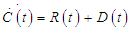where R(t) = recharge rate and D(t) = discharge rate. For example, PB(t) is the net energy available for the battery, with the understanding that PB(t)>0 means the rechsrge cycle is in progress and PB(t)<0 is the discharge cycle is inprogress.
The recharge rate is given by the following model: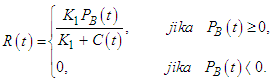Where K2 is a risk factor, operational in the field the value of K2 1.4 which means that only about 70% of the energy stored in the battery can be used to supply the system load. Note that K1 and K2 are assumed to use lead-acid batteries . Since the battery is considered as part of the system, PB(t) becomes a function that depends on other system components and the system load, in this case how much windmill dynamo capacity is used and how much lamp load is used so as to achieve efficient system operational costs. So far the battery model has not affected the conversion from DC to AC (and vice versa).

### 3. Hybrid System Power Generation Configuration

All In general, the hybrid system power plant (HSPP) configuration has components as described in the previous section [3,4,5,6,7,8,9,10,11,12], plus renewable sources of energy, such as windmill generators or micro-hydro power plants. In the HSPP-Parallel Topology configuration, the inverter and the rectifier can be combined into one, which is a two-way inverter (a be-direction inverter) . Thus this configuration is more efficient, because the AC generator can directly supply the demand load without losing energy due to going through the inverter first . In this work we focus only on the HSPP-parallel topology configuration as shown in Figure 3.1.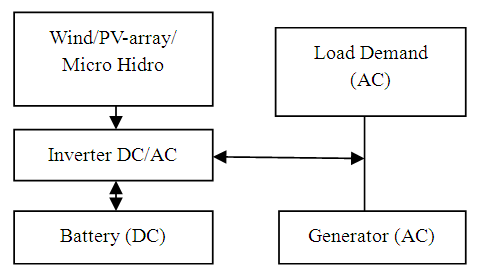Figure 3.1
The capacity of the windmill dynamo and battery is smaller or larger than the values listed in the table can be calculated. The windmill and battery dynamo system is very useful for lighting plantation areas or remote areas that are far from a power source or where the State Electricity Company (PLN) electricity network has not been installed. Approximation of power or capacity of each component of the windmill dynamo system and battery by providing maximum power and life is listed in the table. The electricity supply for remote areas needs to be developed in a sustainable manner with a touch of science and technology that is environmentally friendly. Renewable energy sources (solar, wind, water flow, gravity, …) will be a potential source to be utilized so that it can produce electrical energy.
 Table Approximation of the system unit capacity of the battery and wind-dynamo (12 Volts)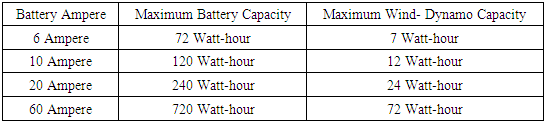The energy produced by the windmill dynamo during the day can be used directly to serve the demand load or stored in batteries, and the energy stored in the battery can be used for lighting at night. The following is a table of correspondence for the use of lamp load capacity (in Watts) and battery capacity so that it can be lit for 12 hours for lighting at night.
 Table Approximate battery capacity and lamp power capacity (12 Volts) for 12 hours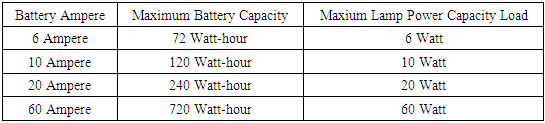K Sunlight as a renewable renewable energy source is one of the energy sources currently in Indonesia that really needs to be developed, given the increasing demand for electrical energy, this is in line with the increasingly limited energy sources from fossil fuels. One of the sources of energy from sunlight is used to generate electricity. Therefore, electricity procurement for remote areas or in urban areas needs to be developed in a sustainable manner with a touch of science and technology that is environmentally friendly.
The next step is to record data every certain time interval of the voltage generated by the solar panels. In this research, data is taken or data is recorded on an electric voltage meter every 1 minute from 07.06 to 17.34 Jakarta time zone on 10 October. Plot the data obtained by the measuring instrument at Cartesian coordinates using Microsoft Excel software which is shown in the following graph.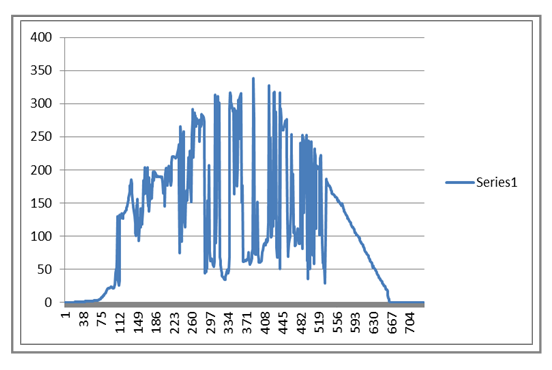Figure 4.1. Plot of sun intensity data from sunrise–sunset obtained on October-10
For data that fluctuates, it is very difficult to make a mathematical model, therefore for the operation of a hybrid system, data from day to day must be interpolated as an external input to the system and the mathematical model obtained applies to large amounts of data (data collected from year to year), or normal shape. However, there is data that is collected in just a day if it is plotted it will produce a graph that is close to the normal curve. This happened because it was a sunny day and the sunlight produced normal power, as shown in the following figure: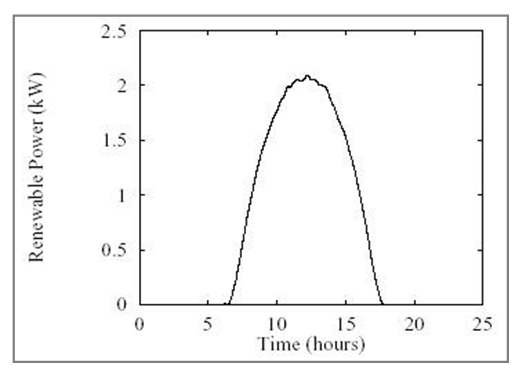Figure 4.2. Plot of solar intensity data that forms a normal curve
The solar intensity power profile (clear weather) from the dataloger which is close to the normal curve is taken as a mathematical model because this condition shows the maximum solar power output. The resulting normal curve is used as a mathematical model for the intensity of the sun from sunrise to sunset. Mathematical modeling of the curve as a function of solar panel power is
Pp = Pmax/108 [(t-6)3 - 4(t-9)3 + 6(t-12)3 – 4(t-15)3 + (t-18)3]. Where Pp(t) is the amount of power generated by the solar panel at t, t is the time from sunrise to sunset and Pmax is the maximum power of the solar panel. The function or model is a normal model obtained from data on sunny weather conditions, sunlight hitting the solar panel from sunrise to sunset.

### 5. Genset Fuel Consumption Model

First of all the generator is off or at an output level of 0% of maximum capacity, this condition is a condition where the generator does not operate. By installing a transparent measuring tube in the fuel tank, the amount of fuel used will be measured. Furthermore, after the generator is ready to operate, install the output power level of 20% of its maximum capacity, by shifting the direction of the needle on the generator engine. For 1 hour the generator is turned on at that level, the amount of fuel used can be measured by observing the measuring tube that has been installed, at that output level the generator can spend about 500 ml of fuel, with energy released 10 kw, at this level the amount of fuel consumed expended relatively little, according to the energy produced.
The approximate power or output capacity of the generator with the amount of fuel every 1 hour is given in the following table:
 Table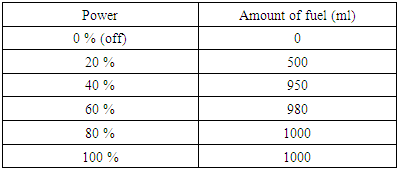Table 5.1 Power level data with the amount of fuel used every 1 hour. Furthermore, the data obtained, plotted using Microsoft excel software and obtained the following graph: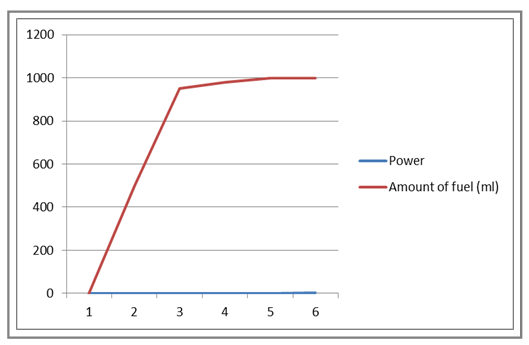Figure
And the graph is transformed into a cost function graph, which is obtained as follows: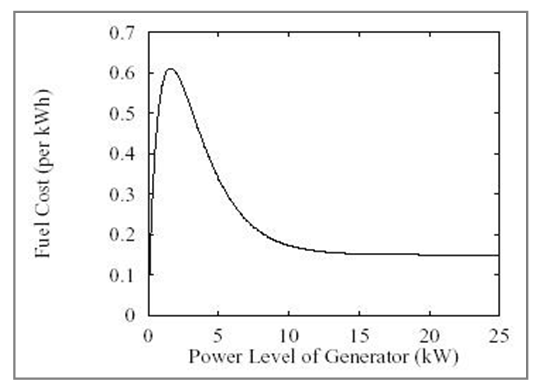Figure 5.1. Graph of the relationship between generator power levels and fuel prices
The following models obtained from existing data.
g1(x) = 2((0,2x + 0,5)0,4 – (0,5)0,4)e-0,1x + 0.15(1 – e-0,1x)). Where x is the generator power level (kw), and g1(x) is the fuel cost.

### 6. Hybrid System Power Plant Operational Strategy

There are many ways to operate a hybrid system as described in. In order for the operational cost of the hybrid system to be efficient, an optimization study is needed to obtain a strategic solution for the operation of the system. Optimization studies need to be carried out because they will provide an accurate solution in designing and operating a hybrid system, so as to achieve the most efficient operational costs. In this study, build a dynamic system model such that the overall operational costs of the system are efficient:
1. If the demand load is less than or equal to the power generated by the generator, the inverter will continue the remaining energy from the generator, windmill or solar panels to charge the battery.
2. If the demand load is less than or equal to the power generated from the combined generator set, windmill and solar panels, but the demand load is greater than the power generated by the generator set, then some of the energy from the turbine and solar panels is used to meet the demand load while the rest is used to charge battery.
3. If the demand load exceeds the power generated from the combined generator, windmill and solar panels, then the battery together with other units supply energy to meet the demand load.
From the steps described above, the operational mathematical model of hybrid system power plant so that the overall operational costs of the system are efficient as follows:
For example, PB(t) is the net electrical energy in the battery, PP(t) is the energy produced by solar panels, PK(t) is the energy produced by the windmill, PG(t) is the energy produced by the generator and PL(t) is the demand load. Furthermore, the three operating options above can be modeled mathematically as follows: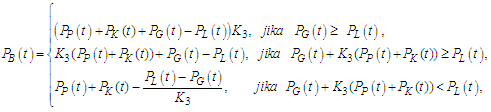where K3 is the inverter efficiency constant.
PB(t) positive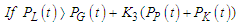and PB(t) negative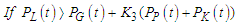with the dynamic model of the system, namely dynamic recharge and discharge of batteries, no energy is wasted. Next, combining the PB(t) expression with the dynamic charge of the battery, we get a dynamic model of the whole hybrid system, namely: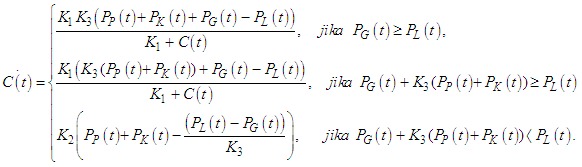In practice the generator can be operated at one of a limited number of output levels, for numerical simulation, the generator control function is assumed that the generator can be operated at an output level of 0% (off), 40% (low), 60% (medium), 80% ( high) and 100% (maximum) of the maximum capacity . The proposed objective function consists of three cost factors. Mathematically, it can be modeled as follows:
1. Generator operational costs are given by model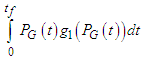with
PG(t) = level output generator in t,
0 ≤ PG(t) ∈ {0%, 40%, 60%, 80%, 100%} ≤ Tmax
g1(PG(t)) = generator fuel cost efficiency function at time t,
g1(x) = 2((0.2x + 0.5)0.4 – (0.5)0.4)e-0.1x + 0.15(1 – e-0.1x)).
2. Charges for recharge and discharge of batteries are given by model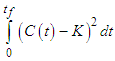with
C(t) = battery capacity at the time t,
K = constant, keep the battery capacity 80% of the maximum capacity,
3. Cost of generator fines for not operating at short time intervals given the model: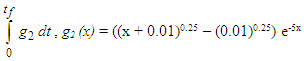### 7. Numerical Results and Discusstion

Some examples of case studies are shown with a 24-hour demand load profile as shown in Figure 7.1 “LOAD”. Battery capacity is limited between Cmin = 20 kWh and Cmax = 100 kWh. Several Co and Ct conditions are given for simulation: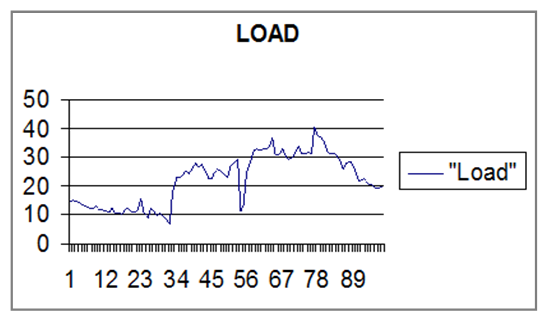Figure 7.1. “LOAD”
Case 1: Co = 80 and Ct = 80. Figure 7.2 shows the optimal charging level, generator level and operating time.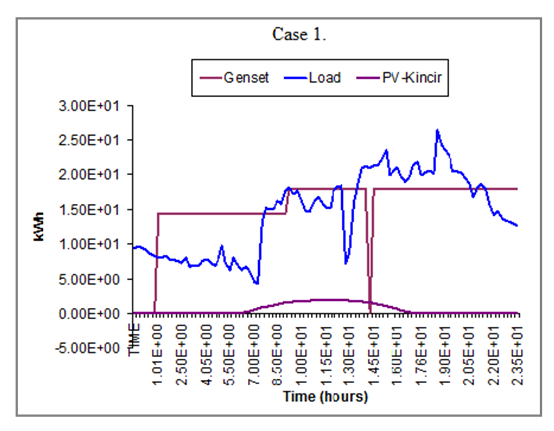Figure 7.2. “Case 1”
Case 2: Co = 95 and Ct = 80. Figure 7.3 shows the optimal charging level, generator level and operating time.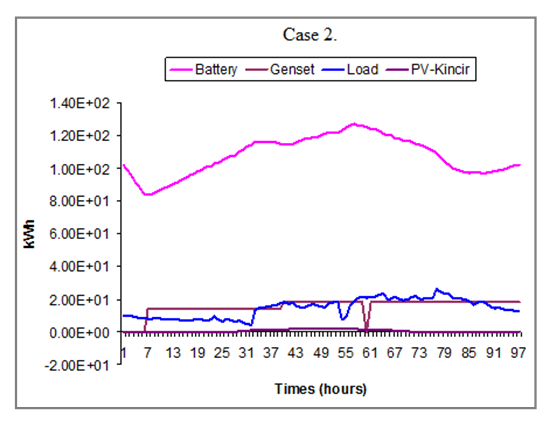Figure 7.3. “Case 2”
Case 3: In this case it is set that the generator does not operate from midnight to 4 am. In practice, this generator operating time limit is very common in the community for reasons of environmental friendliness from noise for communities close to the generator location. Given Co = 90 and Ct = 80. Figure 7.4 shows the optimal charging level, generator level and operating time.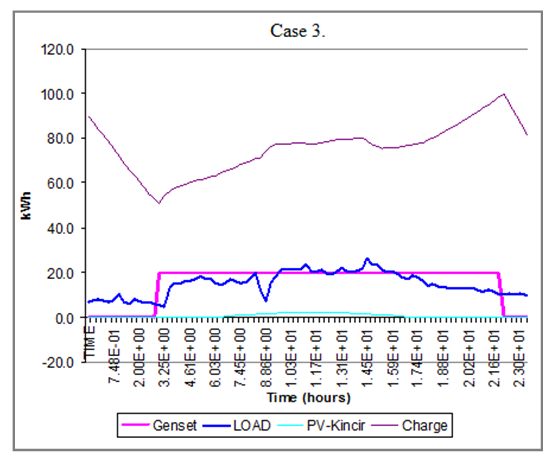Figure 7.4. “Case 3”

### 8. Conclusions

In this paper, we have modelled and developed control stategy for a electricity hybrid systems base on renewable sources using photocell, motion sensor and or wifi switch. Numerical results of the simulation model were presented. The profile recharge and discharge dynamics of lead acid battery as battery bank have shown. The strategy of using photocells, motion sensors and or wifi switch is very relevant in the situation of the pandemic and post-civid-19 pandemic era. the control stategy policies obstained from this model can aid in the future design of better adaptive control strategies.

### ACKNOWLEDGEMENTS

I would like to acknowledge to a number of people for their support and assitance provided during the time I have been working on this research, and all of those who have advised and helped me in the preparation and writing of this paper to be presented in the conference.
Ir. Machudor Yusman, M.Kom, Departement Computer Science, FMIPA Lampung University for being friendly who always available for discussions;
Professor Dr. Karomani, M.Si. as a Rektor and Dr. Ir. Lusmeilia Afriani, D.E.A. as the head of Research Center, University of Lampung, for their willing me to get financial support of BLU Terapan Universitas Lampung, also to the committee of the conference during my participation of LPPM Semnar 2021.

### References

  Harijono T., 2020. Menerangi Negeri: PLN 75 Tahun, Penerbit Buku Kompas PLN.  David Linden and Thomas B. Reddy 2002. Hanbook of Batteries Third Edition (eds.), McGraw-Hill, Two Penn Plaza, New York, NY.  Ashari M. dan Nayar C.V.m 1999. An Optimal Dispatch Strategy Using Set Points for a Photovoltaic (PV)-Diesel-Batteray Hybrid Power System, Solar Energy, Vol. 66 No. 1., pp1-9.  Ashari M. 1997. Optimization of Photovoltaic/Diesel/Battery Hybrid Power Systems for Remote Area Electrification, Masters Thesis, Curtin University of Technology.  David Linden and Thomas B. Reddy 2002. Hanbook of Batteries Third Edition (eds.), McGraw-Hill, Two Penn Plaza, New York, NY.  Manthos Papamattheou dan David Infield. 2000. A New Model for Performance Evaluation of Hybrid Power Systems and its Application to Mykonos, International Journal of Renewable Energy Engineering, Vol. 2 No. 2, pp176-183.  Nayar C.V., Phillips S.J., James W.L., Pryor T.L. and Remmer D. 1993. Novel Wind/Diesel/Battery Hybrid Energy System, Solar Energy, Vol. 51. No 1., pp65-78.  Siddhartha Bhatt M. and Sudhir Kumar R. 2000. Performance Analysis Of Solar Photovoltaic Power Plant-Experimaental Results, International Journal of Renewable Energy Engineering, Vol. 2 No. 2, pp184-191.  Wichert B. and Lawrance W.B. 1998. Photovoltaic Resource and Load Demand Forecasting inStand-alone Renewable Energy System, 2nd World Conference on Photovoltaic Solar Energy Conversion, July, Vienna.  Tiryono, R., V. Rehbock and W.B. Lawrance, “Optimal Control of Hybrid Power Systems”, Dynamics of Continuous, Discrete and Impulsive Systems Series B, 10(3), 2003, 429-440. (Citations: 0; Journal Impact Factor: 0.406).  Tiryono, R., W.R. Lee, and V. Rehbock, “Optimal Design and Operation of Hybrid Power Systems”, Proceedings of Industrial Optimization Symposium, Curtin University 2003, (L. Caccetta and V. Rehbock, Editors), 2004, 1, pp. 324-335.  Tiryono, R., and V. Rehbock, “Numerical Solution of Optimal Switching Control Problems”, in Qi et al. (eds.) Optimization and Control with Applications, Springer, Series on Applied Optimization, Vol. 96, Chapter 21, 2005.  Loxton, R.C., K.L. Teo, V. Rehbock and W.K. Ling, 2009. Optimal Switching Instants for a Switched-Capacitor DC/DC Power Converter, Automatica, 45(4), pp. 973-980.  Tiryono, R., and Agus, S., “Mathematical Modeling of Heat Transper in Agricultural Drying Machine Room (Box Dryer)”, Journal of Physics: Conference Series, 2021 J. Phys.: Conf. Ser. 1751 012029. IOP Publishing.  Lee, W.R., L. Caccetta and V. Rehbock, 2008. Optimal Design of All-Pass Variable Fractional-Delay Digital Filters, DIEEE Transactions on Circuits and Systems I, 55(5), pp. 1248-1256.